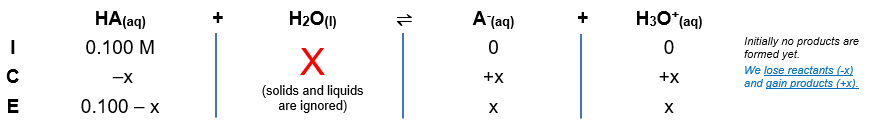# Problem: Calculate the pH at the following point in a titration of 40 mL (0.040 L) of 0.100 M barbituric acid (Ka = 9.8 × 10−5) with 0.100 M KOH.(a) no KOH added

🤓 Based on our data, we think this question is relevant for Professor Price's class at GVSU.

###### FREE Expert Solution

One Ka → monoprotic acid

let HA → barbituric acid

Equilibrium reaction:        HA(aq) + H2O(l)  A-(aq) + H3O+(aq)

Step 1: Construct an ICE chart for the reaction.Step 2: Write the Ka expression.

${\mathbf{K}}_{{\mathbf{a}}}\mathbf{=}\frac{\mathbf{products}}{\mathbf{reactants}}\phantom{\rule{0ex}{0ex}}{\mathbf{K}}_{\mathbf{a}}\mathbf{=}\frac{\mathbf{\left[}{\mathbf{A}}^{\mathbf{-}}\mathbf{\right]}\mathbf{\left[}{\mathbf{H}}_{\mathbf{3}}{\mathbf{O}}^{\mathbf{+}}\mathbf{\right]}}{\left[\mathrm{HA}\right]}$

Solids and liquids are not included in the expression

Step 3: Calculate the equilibrium concentrations.

${\mathbf{K}}_{\mathbf{a}}\mathbf{=}\frac{\left[\mathbf{x}\right]\left[\mathbf{x}\right]}{\left[\mathbf{0}\mathbf{.}\mathbf{100}\mathbf{-}\mathbf{x}\right]}\phantom{\rule{0ex}{0ex}}\mathbf{9}\mathbf{.}\mathbf{8}\mathbf{×}{\mathbf{10}}^{\mathbf{-}\mathbf{5}}\mathbf{=}\frac{{\mathbf{x}}^{\mathbf{2}}}{\mathbf{0}\mathbf{.}\mathbf{100}\mathbf{-}\mathbf{x}}$###### Problem Details

Calculate the pH at the following point in a titration of 40 mL (0.040 L) of 0.100 M barbituric acid (Ka = 9.8 × 10−5) with 0.100 M KOH.